The cubical quad or quad loop is a square-shaped antenna. The gain of a freespace dipole with typical negligible copper losses is 2.13 dBi. Freespace quad element isotropic referenced gain is 3.27 dBi. Thus the quad element has 3.27 - 2.13 =  1.14 dBd  gain over the dipole. We call this 1.14 dBd gain.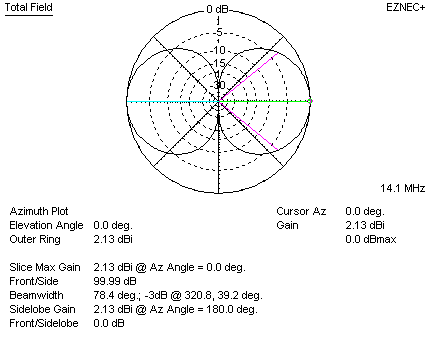Dipole gain freespace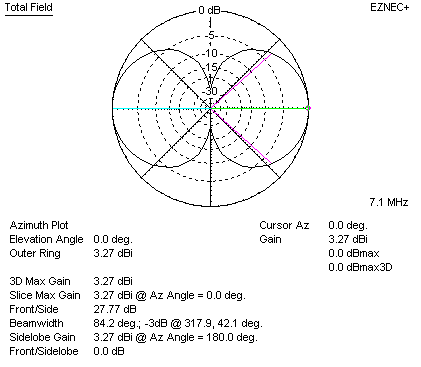The quad element is actually two stacked, shortened, bent dipoles fed in phase. The fed dipole  feeds power to the unfed half through the attached ends. The square shape provides 1/4-wavelength broadside spacing between the two short dipole's current maxima. This more than offsets the immeasurable gain decrease caused by bending the "dipole" antenna. The maximum possible gain of a square quad element over a dipole at the same mean height is just over 1 dB. This peak gain difference occurs in freespace or at an optimum "sweet" height. The "sweet" heights occurs where the dipole pattern has a strong broad direct overhead lobe at heights around .75 wavelength. The quad has no gain over a dipole, and actually can have loss, at heights where the dipole has a vertical pattern null, such as 1/2 and 1 wavelength. The standard horizontally polarized quad element is less effective than a dipole at mean heights below 1/2 wave.

Stacking Gain Graph

From this we see gain of two current maximas spaced .25 wavelengths is about 1 dB over a single element by itself. This agrees with models.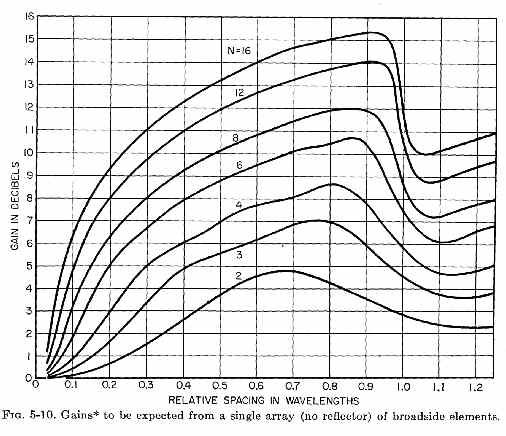## Delta Loop

The delta loop is nothing more and nothing less than a reshaped quad element. The delta is pulled to a point at one side, generally the apex. This either angles one of the high current area's angles to 120 degrees, which further reduces quad element gain, or it makes two bends in high current areas (typically when vertically polarized).

With a single apex point, the delta loop requires only one tall support. The feedpoint, where the heavy coaxial line connects, can be placed at or near the delta loop antenna bottom. The single tall support and low feed line connection point makes delta loop construction and installation exceptionally simple and fast.

Unfortunately, the delta has a pulled-in shape that reduces effective current maxima area and current maxima spacing. The delta loop gives up some of the quad's 1 to 1.2 dB maximum possible gain over a dipole: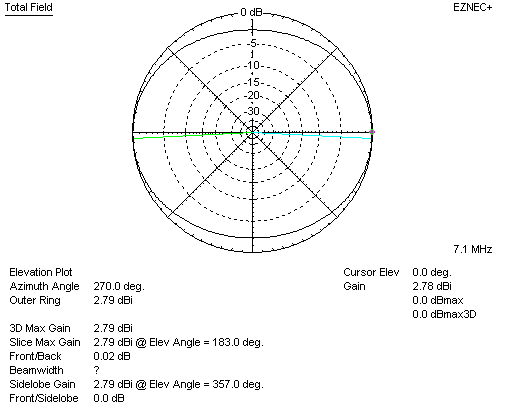The delta loop has 2.78 dBi gain, which is 0.65 dBd gain, in freespace. Freespace is the point of maximum delta gain advantage over a dipole at the same mean height. That gain advantage is 0.65 dB.The quad element, on the other hand, has 3.27 dBi gain, which is 1.14 dBd gain, in freespace.

## Delta Loop vs. Dipole Over Earth, with fixed support height

Let's assume we have a typical 70-foot tower or trees, allowing a maximum height of 70 feet over typical flat, clear, earth. A dipole and delta loop have the following pattern and gain: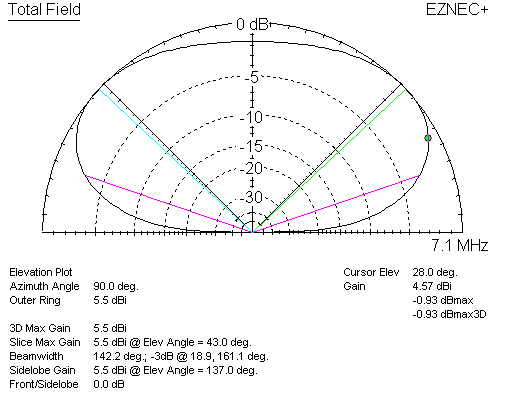At 28 degrees elevation angle, the dipole has 8.09 dBi gain. At the same 28 degree elevation angle, the delta loop has 4.57 dBi gain.  Even at delta loop's maximum gain angle of 43 degrees, the delta loop has only 5.5 dBi gain.

• Compared to an inverted Vee dipole, or dipole, the delta loop lowers effective height of the antenna considerably, increasing wave angle
• The more compact area of the triangular-shaped full wave loop further reduces the already small gain available from a full wave loop

Flattened Delta Loop

The Delta loop or quad loop effective operating height is really the mean height of current maxima locations. With a 70-foot tall support, a delta or quad loop's antenna elevation pattern is one of much lower actual height. Often, because height is limited, delta's are installed in a "squashed" form. This further reduces the maximum available gain of 0.65 dB over a dipole. The delta starts to behave increasingly like a simple folded dipole as it is increasingly squashed. It eventually becomes a folded dipole.

This is a delta squashed to 0.15 wavelength apex to base line height difference.  It has "horizontal polarization" (complimentary current maxima in straight areas, apex feed) in freespace has about 0.1dBd gain: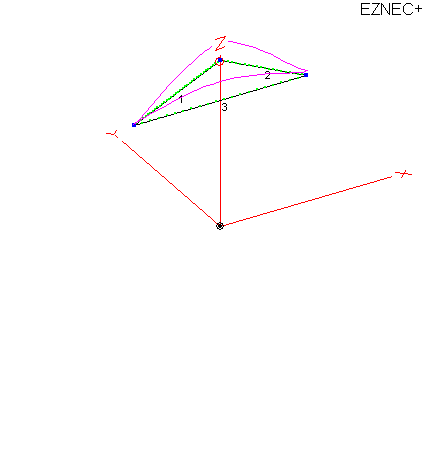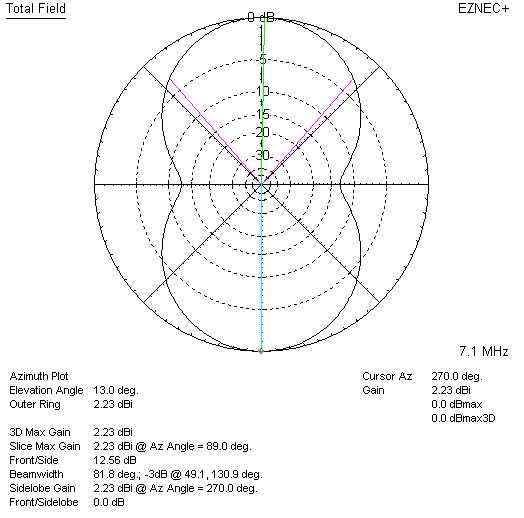## Vertically Polarized Delta LoopFeeding the Delta slightly up from the corner, around 1/4 of the side length up, results in vertical polarization.

Note the current minimum is at the apex, and in the middle of the bottom.

The often used "corner feed" skews the current and polarizations.

Feeding the delta loop on a sloped wire about 25% up either side from a bottom corner produces the following vertically-polarized pattern: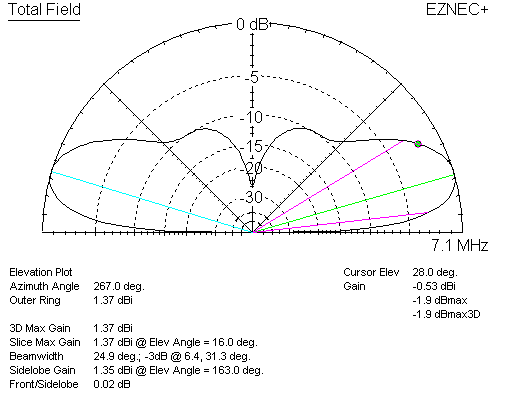At 28 degrees elevation, the delta loop has -.53 dBi gain. This is -8.62 dBd gain, or almost 9 dB weaker than a dipole.

At 16 degrees elevation, the vertically polarized delta has 1.37 dBi gain. When compared to a horizontally polarized dipole at the same maximum height, the delta has -4.72 dBd. Even at peak gain angle of the vertically polarized delta, the horizontally polarized dipole still has more gain.

The vertically polarized delta does not equal the dipole until around 5 degrees elevation angle.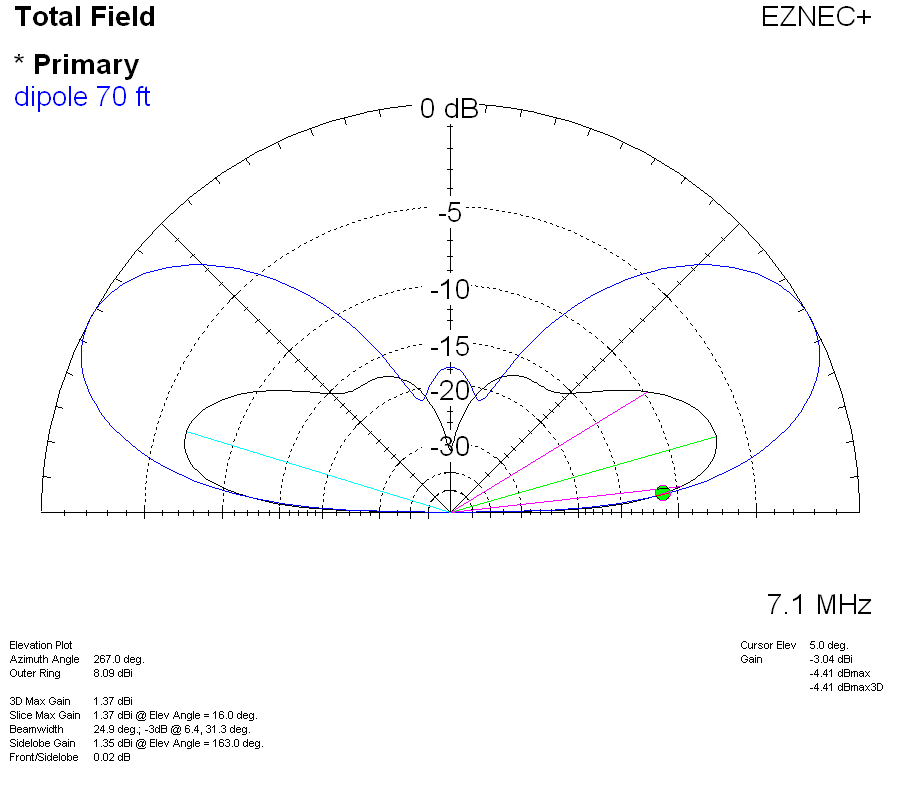Antennas radiate because of current over linear spatial distance. Antennas obtain gain through proper spacing and phase of current maximums.

1.) When antennas are folded in a manner decreasing current maximum spacing, or decreasing the linear (in line) spatial distance of high current area, we reduce gain.

2.) When antennas are installed above earth, effective antenna height is somewhere between peak height and minimum height of high current areas.

3.) These effects apply to all antennas, and to arrays of elements in more complex antennas.